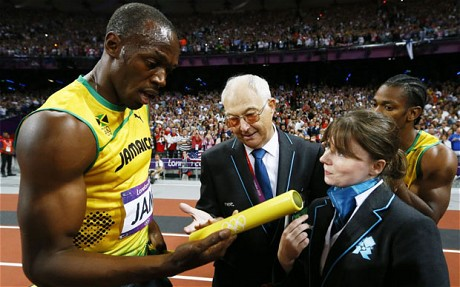Probability Level 4A baton is divided into $2n+1$ cylindrical bands of equal length $(n \geq 1)$. In how many different ways can the $2n+1$ bands be colored if $3$ colors are available, assuming that no two adjacent bands may be given the same color? (Two colorings count as the same if one of them can be converted into the other by turning the baton around.)

This problem is not original. It is on the level of the BMO (British Mathematical Olympiad), and is therefore quite hard.

This problem is part of this set.

×